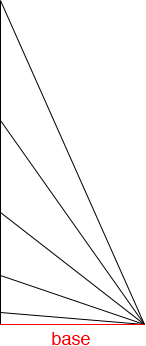SEARCH HOMEMath Central Quandaries & QueriesQuestion from PRAIMNAUTH, a parent: In a right angle triangle , knowing one angle (90 degree ) and the length of the base, how can you calculate the height and the hypotenuse ?Hi,

You can construct many different right angle triangles with the same base. The height can be any positive number and the length of the hypotenuse can be any number larger than the length of the base.PennyMath Central is supported by the University of Regina and The Pacific Institute for the Mathematical Sciences.